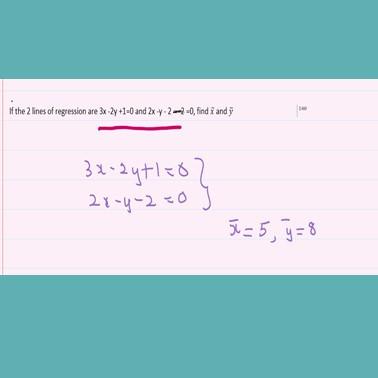Search

# How to Avoid the Standard Mistakes in Regression Analysis

## The course starts with teaching you the difference between dependent and independent variable.Basic Formulas in Regression Analysis

## The relationships between the regression and correlation coefficients is also explained.Note This important tip in Regression Analysis

## Learn this simple problem on RegressionThe 2 lines of Regression intersect at the means

## What happens if the values of x and y are very large? Then we take an assumed mean for x and y and proceed.Calculating Regression coefficients with assumed means